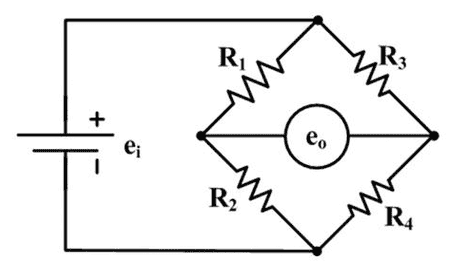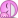# Wheatstone Bridge at Null position

• issacnewton

## Homework Statement

Consider the Wheatstone bridge as shown in the following figure, where ##e_0## is the Galvanometer reading. The condition for ##e_0 = 0 ## is called the null condition of the bridge. Using the Kirchhoff's rules, we can show that the condition is ## R_1R_4 = R_2 R_3##. Now I am trying to prove that if we interchange the emf and the galvanometer at the null position, then the current through the galvanometer is zero.

## Homework Equations

Kirchhoff's rules

## The Attempt at a Solution

Let ##I_i## be the current through the resistance ##R_i##. Now using the Kirchhoff's rules, we can write the following equations.$$I_1 R_1 + I_3 R_3 = I_2 R_2 + I_4 R_4$$
Now I have to exploit the null condition, ## R_1R_4 = R_2 R_3## to prove that the top and bottom point is at the same potential. Then the current through the galvanometer would be zero. But after lot of algebra I am unable to do that. Can anybody guide here ?

thanks#### Attachments

Turn the square with the resistances 1/4 turn while leaving the galvanometer and the battery in place. Now, what is the new condition for i = 0 ? Compare that with the old oneYour math would become a bit simpler if you work with two currents instead of four. You derive one equation, but do not use ##e_0 = 0##

Turn the square with the resistances 1/4 turn while leaving the galvanometer and the battery in place. Now, what is the new condition for i = 0 ? Compare that with the old oneI don't get your point here. What do you mean 1/4 turn ?

90 degrees. r1 ends up in the place where r3 was, etc.

But wouldn't that change the problem itself ?

It is equivalent to interchanging the galvanometer and the battery, as per the exercise you set up for yourself

Well, I am only exchanging the emf and the galvanometer at the null position. So I don't see any equivalence.

You have a certain relationship at this null position. What would be the condition for the 'new' ##e_0 = 0## in the 'new configuration' ?

Well, I can't assume that ##e_0 = 0## in the new configuration. I have to prove it using the null condition achieved in the previous configuration. May be the problem statement was not clear. So one way to prove it would be prove that top point and the bottom point are at the same potential (in the new configuration)

I can't assume
That is correct, and I understand that. That is why I ask what the condition is for new ##e_0=0## .

The condition is that the top point and the bottom point are at the same potential (in the new set up)

I mean in terms of the R

Okay, in terms of resistors, that would mean that ##I_1 R_1 = I_2 R_2##. If I can prove this then it means that top and bottom point are at the same potential.

The condition is that the top point and the bottom point are at the same potential (in the new set up)

But for time being, can I solve the problem using Kirchhoff's rules ?

Yes, if you take the null condition for grantedBut I did play with the null condition ##R_1 R_4 = R_2 R_3## and the equation I have written and I can't come to the conclusion that ##I_1R_1 = I_2 R_2##. Is the mat very hard here or am I missing some aspect ?

But I did play with the null condition ##R_1 R_4 = R_2 R_3## and the equation I have written and I can't come to the conclusion that ##I_1R_1 = I_2 R_2##. Is the mat very hard here or am I missing some aspect ?
What exactly do you want to prove?
Is it R1R4=R2R3 (for null deflection)or I1R1=I2R2 for R1R4=R2R3 ?

Last edited:
Okay, in terms of resistors, that would mean that ##I_1 R_1 = I_2 R_2##. If I can prove this then it means that top and bottom point are at the same potential.
Not good. The potentials the galvanometer 'sees' do not have to be the same in the two configuration. The only requirement is that within a configuration both wires of the galvanpometer see the same potential.

But the top and the bottom point are connected to to the galvanometer in the new configuration. So what else am I supposed to prove here ?

You wanted to prove for yourself that if the bridge is in equilibrium (##e_0=0##), interchanging the connections of battery and galvanometer maintains equilibrium.

Yes, but I seem to be stuck with equations and don't know where to go. I needed help with the equations. I think I am on right track, just stuck with equations.

It is staring you in the face. read all the hints you received

I don't see the hints. I have tried in different ways. Can you be more specific ?

Not more so than in post #2. Did you answer the question ?

Okay, after rotating through 90 degrees in clockwise direction, we reach the configuration which is exactly same as the original one if we had switched emf and galvanometer. And again the condition of no current through the galvanometer, for the rotated configuration is ##R_1 R_4 = R_2 R_3##. This one is same as for the original configuration. So this means that the there will be no current through the galvanometer even after we switched emf and galvanometer at the null point of the galvanometer. So there is no need to do cumbersome algebra here. By symmetry we can arrive at the solution. Your #2 was little confusing but I made sense out of it. But I was wondering if we could reach the same conclusion by setting up equations like I did. I just got lost in the algebra.

I see.
You did not post your
But after lot of algebra I am unable to do that.
load of algebra, so i can't see what goes wrong.

In post #1 you have two equations and eight variables, so I wonder which equations you want to add or which variables you want to consider known.

You might want to add something like $$e_0 = 0 \Rightarrow {R_1\over R_1+R_2} = {R_3\over R_3+R_4}$$ and check if you can prove $${R_1\over R_1+R_2} = {R_3\over R_3+R_4}\Rightarrow {R_1\over R_1+R_3} = {R_2\over R_2+R_4}$$ shouldn't be so hard.

With equivalent rotated configuration, it is exactly the same condition. So there is nothing to solve further. It was great insight into the problem.

Thanks

But I was wondering if we could reach the same conclusion by setting up equations like I did. I just got lost in the algebra
So there is nothing to solve further.
Now I'm lost. I thought you wanted a helping hand out of the algebra, so in #28 I tried to get an idea of where you got stuck.

Well, it clicked to me that your approach of using the symmetry is much better. Symmetry plays very important role in solving physics problems. Thanks again.